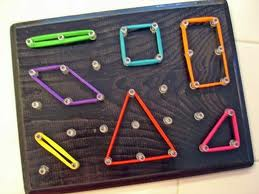1. What’s included when teaching area in 3rd grade?
• Solving real-world problems involving area of polygons
• Solve Real World Problems – Determining when it is necessary to find the area or perimeter from a given story problem.
• Recognize that area is comprised of square units
• Cover a space to determine the area without overlapping or gaps
• Measure area by counting unit squares
• Relate area to multiplication and addition (The formula for area is not addressed until 4th grade but they can relate to arrays for multiplication).
• Find the area of rectilinear figures (shapes with multiple rectangles).

2. Strategies for determining area:
• Give students a problem scenario where they determine which shape covers the most space before telling them there are using square units to determine area. This will help them discover and understand what the concept of area is.

Example: Tony was given the job of sectioning off part of the P.E. court for an assembly. He needs to find the design that will fit the most children. Which rectangle covers more space?• Counting square units
• Use addition/multiplication to determine the area

3. Relate Area to the Real World – Put tasks in real world context and make it relevant. Students should learn how area relates to them in their daily life. This will make it more meaningful and accessible for all students.

Example perimeter problem in real-world context: “Ms. Jones needs to tile this section of the classroom with new square tiles that don’t overlap and have no gaps. How could we determine how many tiles Ms. Jones will need to purchase?”(Note – to make the task even more relevant actually have students explore a given section in the classroom and problem solve).

4. Hands-on Exploration – Use the concrete-representational-abstract format to help students determine and understand the concept of area.

How do you make it happen? Be careful not to rush students to abstract. You want them to have a solid understanding of what area is through hands-on experiences and exploration. Here is a flow that should be utilized:
• Concrete – use manipulatives such as square tile and pentominoes to explore counting units around the outside of a figure to determine the perimeter.
• Representational – use graph paper and pictures to explore area on paper.
• Abstract – determine area of a figure by adding or multiplying. Encourage students to revisit the attributes of different quadrilaterals to make this happen, specifically rectangles and squares. Example: If I know one row in my figure has 5 square tiles and all the rows have the same amount, I can add 5+5+5+5 or multiply 4×5 to find the area of 20 square units.

Fun task to implement – give the students a given are or range of possible areas to look for and have them go around the classroom measuring objects to determine the mystery object(s). Could have them post-it notes or “square units” to determine the area.

Tools to use:
• Square tiles – to represent figures and count to determine area• Pentominoes – to determine area of irregular figures• Geoboards – to create or determine number of square units or area of given polygons• Graph paper – to represent figures and begin using more efficient strategies for determining the area• Rulers – to measure the length of figures to determine the area• Quick pictures – to draw the polygon and determine the area from a given problem

5. Precision with units – make sure students are being precise with their measurement units. Have them label the area in units each time they measure. Also have them compare units: “Would a figure with an area of 20 centimeters or 20 meters be larger?”

Consider using the following book title, “Bigger, Better, Best,” to explore the concept of area!